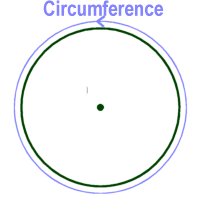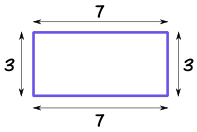# Mathematics Scheme of Work, SS1, 2nd Term

### Scheme of Work (Mathematics) SS1, 2nd Term:

#### WEEK 1

Topic: Factorisation of Quadratic Expression of the form  ax2+bx+c where a, b, c are constants

• Factorising quadratic expression of the form ax2+bx+c
• Factorising quadratic expression of the form ax2-bx+c
• Factorising quadratic expressions of the form ax2+bx-c
• Factorising quadratic expressions of the form ax2-bx-c
• Solving quadratic equation of the form ax2+bx+c = 0 using factorization method.

#### WEEK 2

Topic: Approximation

• Rounding up and rounding down of numbers to significant figures, decimal places and nearest whole numbers.
• Application of approximation to everyday life
• Percentage error.

#### WEEK 3

Topic: Quadratic Equations

• Plotting graph in which one is quadratic function and one is a linear function.
• Using an already plotted curve to find the solution of the various equations.
• Finding the gradient of a curve, the maximum value of y, and minimum value of y and the corresponding values of x.
• Solving a comprehensive quadratic and linear equation graphically.
• Word problem leading to quadratic equations.

#### WEEK 4

Topic: Logical Reasoning

• Meaning of simple statement – open and close statements, true or false.
• Negation of simple statements
• Compound statements – conjunctions, disconjunctions, implication, bi-implication with examples.

#### WEEK 5

Topic: Logical Reasoning

•  Logical operations and symbols – Truth value table – compound statement, Negation (NA), conditional statement, bin-conditional statement.

#### WEEK 6

Topic: Mensuration of Solid Shapes

• Length of arc of a circle with practical demonstration, using formula
• Revision of plane shapes – perimeter of sector and segment
• Area of sector and segment.

#### WEEK 7

Topic: Mensuration of Solid Shapes

• Relationship between the sector of a circle and the surface area of a cone.
• Surface area of solids – cube, cuboids, cylinder, cone, prism, pyramids.

#### WEEK 8

Topic: Mensuration of Solid Shapes

• Volume of solids – cube, cuboids, cylinder, cone, prism, pyramids, frustum of cone and pyramids.
• Surface area and volume of compound shapes.

#### WEEK 9

Topic: Construction

• Lines, line segments, bisection of a line segment e.g. horizontal, vertical, inclined lines etc.
• Construction and bisection of angles e.g. 180o, 90o, 45o, 22o, 60o, 30o, 150o, 75o, 135o, 105o, 165o etc.
• Construction of triangles
• Construction of quadrilaterals.

#### WEEK 10

Topic: Locus of Moving Points

• Equidistant from 2 intersecting straight lines
• Equidistant from 2 points
• Equidistant from a fixed point etc.
• Construction of locus equidistant from a given straight line.

• Revision

#### WEEK 12

• Examination

Mathematics scheme of work, SS1, 1st Term

Mathematics scheme of work, SS1, 3rd Term

Read topics on Mathematics here

THANKS FOR READING - Please Help Share!

#### NOTABLE POINTS IN Mathematics Circumference in mathematics means the distance around the edge of a circle (or a another curverd surface). Perimeter means the distance around a square or rectangle or polygon. Circumference is to circle or any other curved surfaceImage Credit: Ducksters Perimeter is to square or rectangle or polygonImage Credit: Maths is Fun

CONTRIBUTE TO THIS TOPIC | ASK A QUESTION